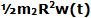## Sunday, February 15, 2009

### Irodov Problem 1.277(a)
Since there is no external torque acting on the (man + disc) system with respect to the axis of rotation, its angular momentum about its axis of rotation with will be conserved at all points in time. Since, the initial angular momentum of the (man + disc) system was zero, so it will be at any given time when the man walks on the edge of the disc. suppose that when the man walks with v'(t) relative to the disc, the disc turns with an angular velocity w(t). The net motion of the man is given by v'(t)+Rw(t). Thus, his angular momentum is given by R[v'(t)+Rw(t)]m1. The angular momentum of the disc is given by(see the list of moment of inertias). Since, net angular velocity of the (man + disc) system must be zero we have,The angle turned can be found by integrating w(t) over time as,s'(t) is the distance traveled by the man along the edge relative to someone on the disc. Since the person walks along the edge the angle walked by the person along the edge is given by. Hence, we have,b) As the man walks he imparts force on the disc and turns it around. We know that,